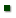Skip to main content

Research Project: Efficient Management and Use of Animal Manure to Protect Human Health and Environmental Quality

Location: Food Animal Environmental Systems Research

Title: Model parameter uncertainty analysis for annual field-scale P loss model

AuthorBolster, CarlVadas, PeterBoykin, Deborah - Debbie

 Submitted to: Journal of Hydrology Publication Type: Peer Reviewed Journal Publication Acceptance Date: 5/3/2016 Publication Date: 5/10/2016 Citation: Bolster, C.H., Vadas, P.A., Boykin, D. 2016. Model parameter uncertainty analysis for annual field-scale P loss model. Journal of Hydrology. 539:27-37. doi: 10.1016/j.jhydrol.2016.05.009. Interpretive Summary: Models are often used to predict phosphorus (P) loss from agricultural fields. While it is commonly recognized that model predictions are inherently uncertain, few studies have addressed prediction uncertainties using P loss models. In this study, we conduct an uncertainty analysis for the Annual P Loss Estimator (APLE) model by estimating the model parameter uncertainty associated with five regression equations used to calculate four of the model variables in APLE. Specifically, we estimate the parameter uncertainties associated with the regression equations used to estimate total soil P from measurements of soil clay content, organic matter, and labile P; the P enrichment ratio from erosion rates; concentration of P in runoff due to labile soil P; and partitioning of P between runoff and infiltration from applied manures and fertilizers. Our analysis included calculating both confidence and prediction intervals. We then calculated predictions of P loss using the APLE model while including uncertainties in both model parameters and model inputs and compared the relative magnitude of these sources of uncertainty to the overall uncertainty associated with predictions of P loss. We also demonstrate how the estimation of model parameter uncertainty can identify model limitations. This study builds on our previous work where we evaluated the effects of model input error on APLE model predictions. Technical Abstract: Phosphorous (P) loss models are important tools for developing and evaluating conservation practices aimed at reducing P losses from agricultural fields. All P loss models, however, have an inherent amount of uncertainty associated with them. In this study, we conducted an uncertainty analysis with the Annual P Loss Estimator (APLE) model, an empirically-based spreadsheet model developed to describe annual, field-scale P loss when surface runoff is the dominant P loss pathway. In particular we evaluated the effects of uncertainties associated with model parameter errors and compared them with uncertainties associated with model inputs. Specifically, we estimated the parameter uncertainties associated with the regression equations used to calculate total soil P from measurements of soil clay content, organic matter, and labile P; the P enrichment ratio determined from erosion rates; concentration of P in runoff calculated from labile soil P; and partitioning of P between runoff and infiltration for applied manures and fertilizers based on runoff ratio. Our analysis included calculating both confidence and prediction intervals. We then calculated predictions of P loss using the APLE model while including uncertainties in both model parameters and inputs and compared the relative magnitude of these sources of uncertainty to the overall uncertainty associated with predictions of P loss. Results from this study highlight the importance of including reasonable estimates of model parameter uncertainties when using models to predict P loss. Our results also demonstrate how the estimation of model parameter uncertainty can identify model limitations.